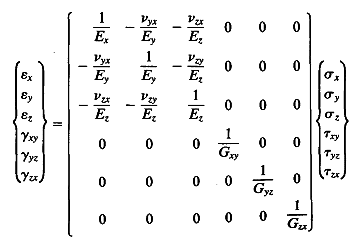# Anisotropic Modulus of elasticity and Poisson's ratio

Hi,
I have some experimental data and I am interested to use this data to calculated modulus of elasticiy (young's modulus) and Poisson's ratio. The material for which the data is given in an anisotropic material, therefore I need to calculate modulus of elasticity and poisson's ration is x,y and z direction.
the data which is have is the stress in three direction and corresponding strain three direction.

So , my problem is simple.
Knows: sigma_x, sigma_y, sigma_z, epsilon_x, epsilon_y, epsilon_y (6 knows)
Unknows: Ex, Ey, Ez, Vxy, Vyx, Vxz (6 unknows).

I have seen a formula in rock mechanics book (see the following image), but it will resolve into only three equation if the share stress is ignored.

Please help me out.
Thanks
Syed## Answers and Replies

Hello Rabbahs, from your post I assume you wish to find the values Ex, Ey, Ez, Vxy, Vyx, and Vxz. For each of the E values, you will need to divide the corresponding sigma value by the corresponding strain value.
In other words, if for example you would like to know Ex, Ex will be equal to σx / εx. This goes for all the E values.

For the poisson values:
Poisson's ratio vxy = - εxy

Bonus: Should you need to calculate the Shear modulus, Gxy = τxy xy.
Or Gx = taux /gammax.

I hope this helps.

Thanks darovmat for you reply, I really appreciate it.
The data which I have is for the deformation in x,y and z direction when sample is compressed in all three direction simultaneously. Therefore in my data the effected of anisotropy is coupled in all three strain value due to difference in poisson's ration in x,y and z direction (material is highly anisotropic).
The formula you have stated is only valid for very simple case when only axial stress is applied and strain in measured.

Please let me know you comments.
thanks
Syed